# DeepHit for Single Event¶

In this notebook we show an example of how we can fit a DeepHit model when we only have one event type.

If you are interested in competing risks, see this notebook instead.

For a more verbose introduction to pycox see this notebook.

In :
import numpy as np
import matplotlib.pyplot as plt

# For preprocessing
from sklearn.preprocessing import StandardScaler
from sklearn_pandas import DataFrameMapper

import torch # For building the networks
import torchtuples as tt # Some useful functions

from pycox.datasets import metabric
from pycox.models import DeepHitSingle
from pycox.evaluation import EvalSurv

In :
## Uncomment to install sklearn-pandas
# ! pip install sklearn-pandas

In :
np.random.seed(1234)
_ = torch.manual_seed(123)


## Dataset¶

We load the METABRIC data set as a pandas DataFrame and split the data in in train, test and validation.

The duration column gives the observed times and the event column contains indicators of whether the observation is an event (1) or a censored observation (0).

In :
df_train = metabric.read_df()
df_test = df_train.sample(frac=0.2)
df_train = df_train.drop(df_test.index)
df_val = df_train.sample(frac=0.2)
df_train = df_train.drop(df_val.index)

In :
df_train.head()

Out:
x0 x1 x2 x3 x4 x5 x6 x7 x8 duration event
0 5.603834 7.811392 10.797988 5.967607 1.0 1.0 0.0 1.0 56.840000 99.333336 0
1 5.284882 9.581043 10.204620 5.664970 1.0 0.0 0.0 1.0 85.940002 95.733330 1
3 6.654017 5.341846 8.646379 5.655888 0.0 0.0 0.0 0.0 66.910004 239.300003 0
4 5.456747 5.339741 10.555724 6.008429 1.0 0.0 0.0 1.0 67.849998 56.933334 1
5 5.425826 6.331182 10.455145 5.749053 1.0 1.0 0.0 1.0 70.519997 123.533333 0

## Feature transforms¶

The METABRIC dataset has 9 covariates: x0, ..., x8. We will standardize the 5 numerical covariates, and leave the binary covariates as is. Note that PyTorch require variables of type 'float32'.

We like using the sklearn_pandas.DataFrameMapper to make feature mappers.

In :
cols_standardize = ['x0', 'x1', 'x2', 'x3', 'x8']
cols_leave = ['x4', 'x5', 'x6', 'x7']

standardize = [([col], StandardScaler()) for col in cols_standardize]
leave = [(col, None) for col in cols_leave]

x_mapper = DataFrameMapper(standardize + leave)

In :
x_train = x_mapper.fit_transform(df_train).astype('float32')
x_val = x_mapper.transform(df_val).astype('float32')
x_test = x_mapper.transform(df_test).astype('float32')


## Label transforms¶

The survival methods require individual label transforms, so we have included a proposed label_transform for each method. In this case label_transform is just a shorthand for the class pycox.preprocessing.label_transforms.LabTransDiscreteTime.

DeepHit is a discrete-time method, meaning it requires discretization of the event times to be applied to continuous-time data. We let num_durations define the size of this (equidistant) discretization grid, meaning our network will have num_durations output nodes.

In :
num_durations = 10
labtrans = DeepHitSingle.label_transform(num_durations)
get_target = lambda df: (df['duration'].values, df['event'].values)
y_train = labtrans.fit_transform(*get_target(df_train))
y_val = labtrans.transform(*get_target(df_val))

train = (x_train, y_train)
val = (x_val, y_val)

# We don't need to transform the test labels
durations_test, events_test = get_target(df_test)

In :
type(labtrans)

Out:
pycox.preprocessing.label_transforms.LabTransDiscreteTime

## Neural net¶

We make a neural net with torch. For simple network structures, we can use the MLPVanilla provided by torchtuples. For building more advanced network architectures, see for example the tutorials by PyTroch.

The following net is an MLP with two hidden layers (with 32 nodes each), ReLU activations, and num_nodes output nodes. We also have batch normalization and dropout between the layers.

In :
in_features = x_train.shape
num_nodes = [32, 32]
out_features = labtrans.out_features
batch_norm = True
dropout = 0.1

net = tt.practical.MLPVanilla(in_features, num_nodes, out_features, batch_norm, dropout)


If you instead want to build this network with torch you can uncomment the following code. It is essentially equivalent to the MLPVanilla, but without the torch.nn.init.kaiming_normal_ weight initialization.

In :
# net = torch.nn.Sequential(
#     torch.nn.Linear(in_features, 32),
#     torch.nn.ReLU(),
#     torch.nn.BatchNorm1d(32),
#     torch.nn.Dropout(0.1),

#     torch.nn.Linear(32, 32),
#     torch.nn.ReLU(),
#     torch.nn.BatchNorm1d(32),
#     torch.nn.Dropout(0.1),

#     torch.nn.Linear(32, out_features)
# )


## Training the model¶

To train the model we need to define an optimizer. You can choose any torch.optim optimizer, but here we instead use one from tt.optim as it has some added functionality. We use the Adam optimizer, but instead of choosing a learning rate, we will use the scheme proposed by Smith 2017 to find a suitable learning rate with model.lr_finder. See this post for an explanation.

We also set duration_index which connects the output nodes of the network the the discretization times. This is only useful for prediction and does not affect the training procedure.

DeepHit has a loss that is a combination of a negative log-likelihood and a ranking loss. alpha is a parameter that controls the linear combination between the two, and sigma is a parameter used by the ranking loss. alpha = 1 give a loss only containing the negative log-likelihood and alpha = 0 give a pure ranking loss. Note that this is different than the original paper.

In :
model = DeepHitSingle(net, tt.optim.Adam, alpha=0.2, sigma=0.1, duration_index=labtrans.cuts)

In :
batch_size = 256
lr_finder = model.lr_finder(x_train, y_train, batch_size, tolerance=3)
_ = lr_finder.plot()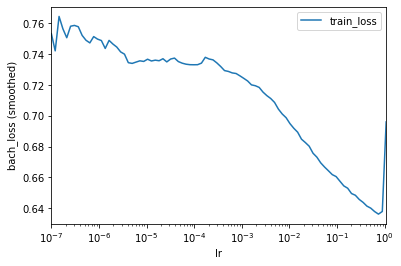In :
lr_finder.get_best_lr()

Out:
0.07390722033525823

Often, this learning rate is a little high, so we instead set it manually to 0.01

In :
model.optimizer.set_lr(0.01)


We include the EarlyStopping callback to stop training when the validation loss stops improving. After training, this callback will also load the best performing model in terms of validation loss.

In :
epochs = 100
callbacks = [tt.callbacks.EarlyStopping()]
log = model.fit(x_train, y_train, batch_size, epochs, callbacks, val_data=val)

0:	[0s / 0s],		train_loss: 0.6729,	val_loss: 0.5150
1:	[0s / 0s],		train_loss: 0.5835,	val_loss: 0.5140
2:	[0s / 0s],		train_loss: 0.5536,	val_loss: 0.5021
3:	[0s / 0s],		train_loss: 0.5422,	val_loss: 0.5063
4:	[0s / 0s],		train_loss: 0.5292,	val_loss: 0.5033
5:	[0s / 0s],		train_loss: 0.5207,	val_loss: 0.5011
6:	[0s / 0s],		train_loss: 0.5101,	val_loss: 0.5014
7:	[0s / 0s],		train_loss: 0.5130,	val_loss: 0.5037
8:	[0s / 0s],		train_loss: 0.5085,	val_loss: 0.4986
9:	[0s / 0s],		train_loss: 0.5069,	val_loss: 0.4990
10:	[0s / 0s],		train_loss: 0.4977,	val_loss: 0.4984
11:	[0s / 0s],		train_loss: 0.4908,	val_loss: 0.5004
12:	[0s / 0s],		train_loss: 0.4952,	val_loss: 0.4988
13:	[0s / 0s],		train_loss: 0.4914,	val_loss: 0.5005
14:	[0s / 0s],		train_loss: 0.4887,	val_loss: 0.5092
15:	[0s / 0s],		train_loss: 0.4846,	val_loss: 0.5100
16:	[0s / 0s],		train_loss: 0.4721,	val_loss: 0.5068
17:	[0s / 0s],		train_loss: 0.4830,	val_loss: 0.5061
18:	[0s / 0s],		train_loss: 0.4831,	val_loss: 0.5063
19:	[0s / 0s],		train_loss: 0.4772,	val_loss: 0.5074
20:	[0s / 1s],		train_loss: 0.4773,	val_loss: 0.5081

In :
_ = log.plot()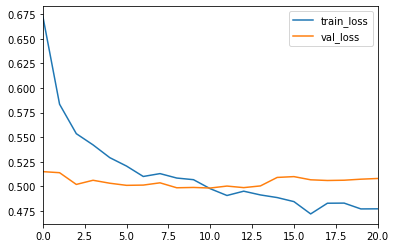## Prediction¶

For evaluation we first need to obtain survival estimates for the test set. This can be done with model.predict_surv which returns an array of survival estimates, or with model.predict_surv_df which returns the survival estimates as a dataframe.

In :
surv = model.predict_surv_df(x_test)


We can plot the survival estimates for the first 5 individuals. Note that the time scale is correct because we have set model.duration_index to be the grid points. We have, however, only defined the survival estimates at the 10 times in our discretization grid, so, the survival estimates is a step function

In :
surv.iloc[:, :5].plot(drawstyle='steps-post')
plt.ylabel('S(t | x)')
_ = plt.xlabel('Time')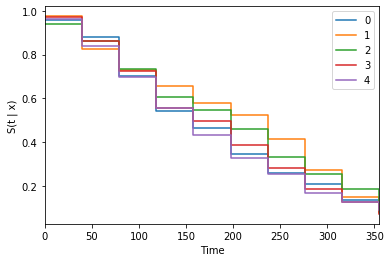It is, therefore, often beneficial to interpolate the survival estimates, see this paper for a discussion. Linear interpolation (constant density interpolation) can be performed with the interpolate method. We also need to choose how many points we want to replace each grid point with. Her we will use 10.

In :
surv = model.interpolate(10).predict_surv_df(x_test)

In :
surv.iloc[:, :5].plot(drawstyle='steps-post')
plt.ylabel('S(t | x)')
_ = plt.xlabel('Time')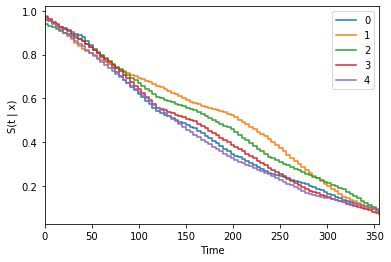## Evaluation¶

The EvalSurv class contains some useful evaluation criteria for time-to-event prediction. We set censor_surv = 'km' to state that we want to use Kaplan-Meier for estimating the censoring distribution.

In :
ev = EvalSurv(surv, durations_test, events_test, censor_surv='km')


#### Concordance¶

In :
ev.concordance_td('antolini')

Out:
0.6556657764212133

#### Brier Score¶

We can plot the the IPCW Brier score for a given set of times. Here we just use 100 time-points between the min and max duration in the test set. Note that the score becomes unstable for the highest times. It is therefore common to disregard the rightmost part of the graph.

In :
time_grid = np.linspace(durations_test.min(), durations_test.max(), 100)
ev.brier_score(time_grid).plot()
plt.ylabel('Brier score')
_ = plt.xlabel('Time')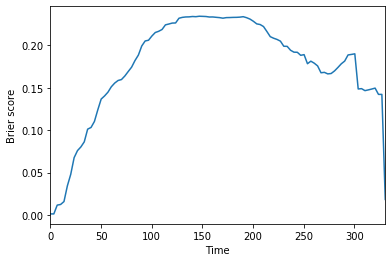#### Negative binomial log-likelihood¶

In a similar manner, we can plot the the IPCW negative binomial log-likelihood.

In :
ev.nbll(time_grid).plot()
plt.ylabel('NBLL')
_ = plt.xlabel('Time')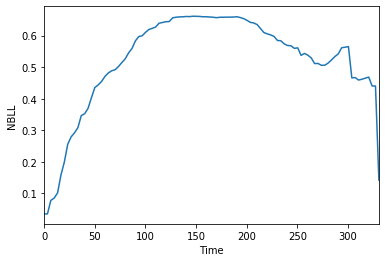#### Integrated scores¶

The two time-dependent scores above can be integrated over time to produce a single score Graf et al. 1999. In practice this is done by numerical integration over a defined time_grid.

In :
ev.integrated_brier_score(time_grid)

Out:
0.17627978358784843
In :
ev.integrated_nbll(time_grid)

Out:
0.5240259319069138
In [ ]: